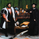TradingView4302 lượt xem
Correlation Based Pair Trading Strategy (Trading the spread)

There are three popular styles of Pair trading:

* Distance based pair trading
* Correlation based pair trading
* Cointegration based pair trading

The correlation based strategy is to short the outperforming instrument and go long on the underperforming one
whenever the temporary correlation weakens which means one instrument going up and another going down.
Here, instead of two different instruments two timeframes of the same instrument are used, lower and higher.
In order to calculate the trade size, we would need to calculate hedge_ratio,
which is simply the ratio of the closing price of the instrument in the current tf to the higher tf.
So whenever we want to go short on spread we’ll short 1 unit of current tf and go long on hedge ratio times units of higher tf.

In order to generate trading signals, we need to define our trading logic.
So, we’ll go long on the spread when the hedge ratio goes below its p rolling mean by mult times of its
rolling standard deviation and vice versa.
Note that both p and mult can be changed as per your selection of instruments.
See: https://analyticsprofile.com/algo-tradin...

NOTE:
There is a minor problem to it in Pinescript. After a while the signal line goes horizontal and the two bounds, upper and lower, converge unless you update the time frame.
Kepp me in the know if you find a solution to this problem)
Phát hành các Ghi chú: Correlation Based Pair Trading Strategy (Trading the spread)

There are three popular styles of Pair trading:

* Distance based pair trading
* Correlation based pair trading
* Cointegration based pair trading

The correlation based strategy is to short the outperforming instrument and go long on the underperforming one
whenever the temporary correlation weakens which means one instrument going up and another going down.
Here, instead of two different instruments two timeframes of the same instrument are used, lower and higher.
In order to calculate the trade size, we would need to calculate hedge_ratio,
which is simply the ratio of the closing price of the instrument in the current tf to the higher tf.
So whenever we want to go short on spread we’ll short 1 unit of current tf and go long on hedge ratio times units of higher tf.

In order to generate trading signals, we need to define our trading logic.
So, we’ll go long on the spread when the hedge ratio goes below its p rolling mean by mult times of its
rolling standard deviation and vice versa.
Note that both p and mult, as well as tf, can be changed as per your selection of instruments.
See: https://analyticsprofile.com/algo-tradin...
Mã nguồn mở

Với tinh thần của TradingView, tác giả đã xuất bản tập lệnh theo mã nguồn mở, vì thế trader có thể dễ dàng hiểu và tùy chỉnh được. Bạn có thể sử dụng miễn phí, hoặc tùy chỉnh lại mã đã được cấp phép bởi Quy tắc Chung. Bạn có thể sử dụng nó trên biểu đồ.

Bạn muốn sử dụng tập lệnh này trên biểu đồ?

## Bình luậnReplaced the line
Y = security(tickerid, customReso, X)
with
Y = security(tickerid, customReso, X, barmerge.gaps_off, barmerge.lookahead_on),
so now the indicator updates as required.
Phản hồi